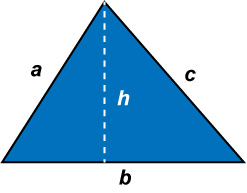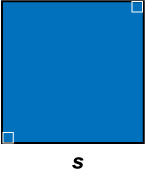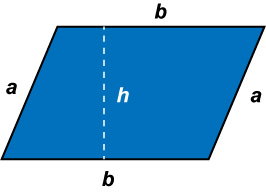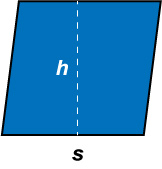# Area and Perimeter Collection

## Review of Area and Perimeter Formulas

### PerimeterTriangleRight TriangleSquareParallelogramRhombusNumber of Resources: 318
Thumbnail Image Description

## Math Definitions Collection: GeometryThis collection aggregates all the definition image cards around the topic of Geometry vocabulary. There are a total of 58 terms.

## Video Tutorial: Perimeter of a TriangleVideo Tutorial: Perimeter of a Triangle

In this video tutorial learn about perimeter in the context of triangles. Several examples of calculating a perimeter are shown: whole number sides, decimal sides. Then we look at triangles one of whose sides is a variable. The resulting perimeter is an algebraic expression.

## Math Example--Area and Perimeter--Area Models for Polynomials: Example 4Math Example--Area and Perimeter--Area Models for Polynomials: Example 4

This is part of a collection of math examples that show how to area and perimeter for different geometric shapes.

## Wildlife RefugeDescription

In this module students use their geometry and algebra skills to analyze a wildlife refuge in Nevada. Calculating the area, perimeter, and the ratio of the two allows students to analyze different configurations for a refuge. Specifically, they look at ways of designing a wild horse refuge, using the concepts they have learned.

## Instructional Resource: TI-Nspire Activity: Constructing and Measuring the Area of a PolygonIn this TI-Nspire Activity, use the Geometry Tools to construct a regular polygon and measure its area using the apothem-perimeter formula.

## Math Worksheet Collection: Using Distance Formula for Area and PerimeterThis collection aggregates all the math worksheets around the topic of Using Distance Formula for Area and Perimeter. There are a total of 140 worksheets.

## Math Examples Collection: Triangular Area and PerimeterOverv
This collection aggregates all the math examples around the topic of Triangular Area and Perimeter. There are a total of 40 Math Examples.

## Math Examples Collection: Rectangular Area with TilesOverview T
This collection aggregates all the math examples around the topic of Rectangular Area with Tiles. There are a total of 6 Math Examples.

## Math Examples Collection: Rectangular Area and PerimeterThis collection aggregates all the math examples around the topic of Rectangular Area and Perimeter. There are a total of 22 Math Examples.

## Math Examples Collection: Quadrilateral Area and PerimeterThis collection aggregates all the math examples around the topic of Quadrilateral Area and Perimeter. There are a total of 24 Math Examples.

## Math Examples Collection: Perfect Squares and Cubes--Area and VolumeThis collection aggregates all the math examples around the topic of Perfect Squares and Cubes--Area and Volume. There are a total of 7 Math Examples.

## Closed Captioned Video: Geometry Applications: Area and VolumeClosed Captioned Video: Geometry Applications: Area and Volume

In this program we look at applications of area and volume. We do this in the context of three real-world applications. In the first, we look at the sinking of the Titanic in the context of volume and density.

## Closed Captioned Video: Geometry Applications: Area and Volume, 1Closed Captioned Video: Geometry Applications: Area and Volume, Segment 1: Volume and Density.

The sinking of the Titanic provides an opportunity to explore volume, density, and buoyancy. Students construct a mathematical model of the Titanic to determine why it sank and what could have been done to prevent it from sinking.

## Closed Captioned Video: Geometry Applications: Area and Volume, 2Closed Captioned Video: Geometry Applications: Area and Volume, Segment 2: Surface Area.

The glass-paneled pyramid at the Louvre Museum in Paris is a tessellation of rhombus-shaped glass panels. Students create a model of the pyramid to calculate the number of panels used to cover the surface area of the pyramid.

## Closed Captioned Video: Geometry Applications: Area and Volume, 3Closed Captioned Video: Geometry Applications: Area and Volume, Segment 3: Ratio of Surface Area to Volume.

The Citibank Tower in New York City presents some unique design challenges. In addition it has to cope with a problem that all tall structure have to deal with: heat loss.

## Math Example--Area and Perimeter--Triangular Area Using Trig Ratios: Example 11Math Example--Area and Perimeter--Triangular Area Using Trig Ratios: Example 11

This is part of a collection of math examples that show how to area and perimeter for different geometric shapes.

## Math Example--Area and Perimeter--Triangular Area Using Trig Ratios: Example 10Math Example--Area and Perimeter--Triangular Area Using Trig Ratios: Example 10

This is part of a collection of math examples that show how to area and perimeter for different geometric shapes.

## Math Example--Area and Perimeter--Triangular Area Using Trig Ratios: Example 9Math Example--Area and Perimeter--Triangular Area Using Trig Ratios: Example 9

This is part of a collection of math examples that show how to area and perimeter for different geometric shapes.

## Math Example--Area and Perimeter--Triangular Area Using Trig Ratios: Example 8Math Example--Area and Perimeter--Triangular Area Using Trig Ratios: Example 8

This is part of a collection of math examples that show how to area and perimeter for different geometric shapes.

## Math Example--Area and Perimeter--Triangular Area Using Trig Ratios: Example 7Math Example--Area and Perimeter--Triangular Area Using Trig Ratios: Example 7

This is part of a collection of math examples that show how to area and perimeter for different geometric shapes.

## Math Example--Area and Perimeter--Triangular Area Using Trig Ratios: Example 6Math Example--Area and Perimeter--Triangular Area Using Trig Ratios: Example 6

This is part of a collection of math examples that show how to area and perimeter for different geometric shapes.

## Math Example--Area and Perimeter--Triangular Area Using Trig Ratios: Example 5Math Example--Area and Perimeter--Triangular Area Using Trig Ratios: Example 5

This is part of a collection of math examples that show how to area and perimeter for different geometric shapes.

## Math Example--Area and Perimeter--Triangular Area Using Trig Ratios: Example 4Math Example--Area and Perimeter--Triangular Area Using Trig Ratios: Example 4

This is part of a collection of math examples that show how to area and perimeter for different geometric shapes.

## Math Example--Area and Perimeter--Triangular Area Using Trig Ratios: Example 3Math Example--Area and Perimeter--Triangular Area Using Trig Ratios: Example 3

This is part of a collection of math examples that show how to area and perimeter for different geometric shapes.

## Math Example--Area and Perimeter--Triangular Area Using Trig Ratios: Example 2Math Example--Area and Perimeter--Triangular Area Using Trig Ratios: Example 2

This is part of a collection of math examples that show how to area and perimeter for different geometric shapes.

## Math Example--Area and Perimeter--Triangular Area Using Trig Ratios: Example 1Math Example--Area and Perimeter--Triangular Area Using Trig Ratios: Example 1

This is part of a collection of math examples that show how to area and perimeter for different geometric shapes.

## Math Example--Polynomial Concepts-- Perfect Squares and Cubes--Example 7Math Example--Polynomial Concepts-- Perfect Squares and Cubes--Example 7

This is part of a collection of math examples that focus on polynomial concepts. This includes adding, subtracting, multiplying, and dividing polynomials, along with polynomial properties.

## Math Example--Polynomial Concepts-- Perfect Squares and Cubes--Example 6Math Example--Polynomial Concepts-- Perfect Squares and Cubes--Example 6

This is part of a collection of math examples that focus on polynomial concepts. This includes adding, subtracting, multiplying, and dividing polynomials, along with polynomial properties.

## Math Example--Polynomial Concepts-- Perfect Squares and Cubes--Example 5Math Example--Polynomial Concepts-- Perfect Squares and Cubes--Example 5

This is part of a collection of math examples that focus on polynomial concepts. This includes adding, subtracting, multiplying, and dividing polynomials, along with polynomial properties.

## Math Example--Polynomial Concepts-- Perfect Squares and Cubes--Example 4Math Example--Polynomial Concepts-- Perfect Squares and Cubes--Example 4

This is part of a collection of math examples that focus on polynomial concepts. This includes adding, subtracting, multiplying, and dividing polynomials, along with polynomial properties.